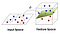# Kernel Regression from scratch in python

Okay, there are already plenty of articles describing linear regression but I have not seen much on kernelized regression. Well the fact that you are reading this means that you also didn’t find what you were looking for…

For completeness we will get started with ordinary linear regression.

# Ordinary Linear Regression

You can think of this data as whatever you want. Here we can clearly see that there is a strong correlation between the two variables. X-Axis could be price of a bus-ticket and y-axis could be the distance traveled, as we would expect a strong correlation there. You’re data likely looks completely different but linear regression works all the same.

Let’s have a closer look at the data. In our case the data X is just one dimensional but we will keep it more general so we have n=100 samples each of dimension d=1.

We know the equation of a line is y=mx. We simply let our data X represent the general x and then we just need to solve for m.

Since we can not invert a matrix that isn’t square we simply make X square by multiplying with its own transpose before inversion. To get the original shape back and by magic of math we multiply by the transpose again and get what’s know as Moore–Penrose inverse.

Okay finally time for more code.

Well we got a line… but it doesn’t really match the data yet. So what went wrong? The line equation that we started with y=mx will always pass through (0,0). No matter what value you pick for m at x=0, y has to be 0. However the regression line we expect from our data does not pass through (0,0). So we have to adjust our line equation so that it’s y-value at x=0 can be fitted by our approach. We all know from school that we can simply add a constant to our line formula to end up with y=mx+b.

How are we going to this with our current equation? Let’s look at what we have so far:

Now if we just add an extra dimension to our data we can notice that the same multiplication of X and m will require m to be 2 dimensional just like each sample in X. And the matrix multiplication looks like this:

Now if we set x[:,1]=1 and we view m as b we get the line equation with our bias b! So we don’t even need to change our equation at all!

That looks much better already!

We are not going to discuss the remaining topics in detail. The rest is just to get you started and give you a general idea.

# Primal vs Dual Form

Now we can compute the inverse just as well by changing the order of the transpose.

This is known as the dual Form. Notice that the matrix that needs to be inverted is know a square matrix of the dimension n x n! This is much more expensive to compute when we have many more samples than dimensions in X. So most of the time we want to avoid this. However it can be useful to derive solutions for classification problems such as SVM so it’s good to have seen this before.

# Kernel RegressionCC BY-SA 4.0Photo by Shehzadex on Wikimedia

What does that transformation look like? Just to give you an idea a simple polynomial mapping could look like this:

Well that certainly look like a lot of work for us and the computer. We went from 2 dimension to 6. Not only do we have to compute that embedding in the first place anything we do from here on has to work on the 6 dimensions rather than 2. Luckily some smart people noticed that there are certain special transformation where we can reduce a dot product in the feature space to a simple evaluation in the original dimension!

Though it might not look like much.This is HUGE. So for example the polynomial function is such a special kernel function (Mercer Kernel). For a simply polynomial kernel the feature map already has a ridiculously large dimension:

We can replace any vector dot-product of this behemoth with a simply function. Even better looking at our linear regression we notice that our data X occurs only in dot-product so we can replace all of them!

Now we just take our by now familiar linear regression and replace all dot products with kernel functions.

Notice that we also have to embed the vector at which we evaluate the regression, here z. Putting this into code we get:

A nice non-linear regression using the kernel trick! For completeness here is the code used for the graphs: